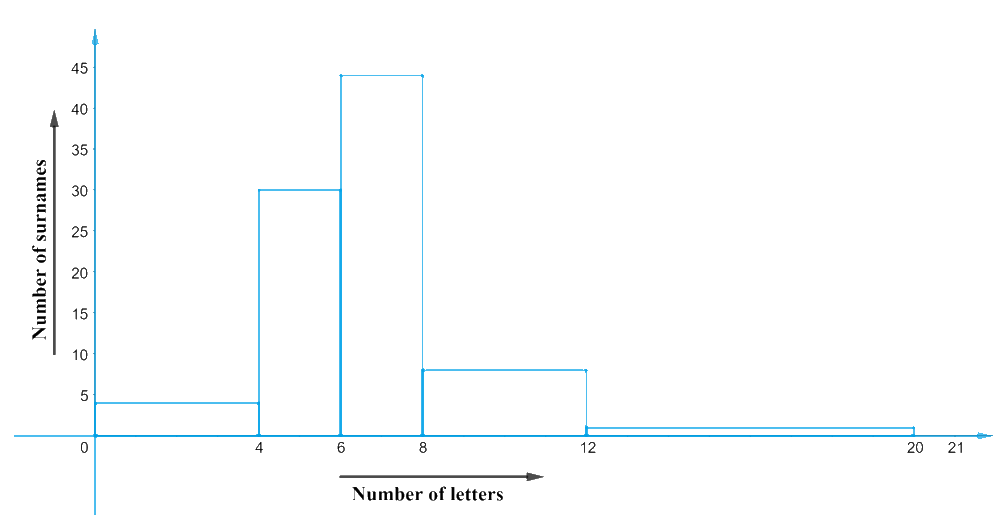# Ex.14.3 Q9 Statistics Solution - NCERT Maths Class 9

Go back to  'Ex.14.3'

## Question

Surnames were randomly picked up from a local telephone directory and a frequency distribution of the number of letters in the English alphabet in the surnames was found as follows:

 Number of letters Number of surnames $$1 – 4$$ $$6$$ $$4 – 6$$ $$30$$ $$6 – 8$$ $$44$$ $$8 – 12$$ $$16$$ $$12 – 20$$ $$4$$

(i) Draw a histogram to depict the given information.

(ii) Write the class interval in which the maximum number of surnames lie.

Video Solution
Statistics
Ex exercise-14-3 | Question 9

## Text Solution

What is known?

Distribution of the number of letters in the English alphabet in the surnames.

What is Unknown?

(i) A Histogram to depict the given data.

(ii) The class interval in which the maximum number of surnames lies.

Reasoning:

(i) It can be observed from the given data that it has class intervals of varying width.

(ii) The proportion of number of surnames per $$2$$ letter interval (class interval of min class size for reference) can be made.

 Number of letters Number of surnames Width of the class Length of rectangle $$1 – 4$$ $$6$$ $$3$$ \begin{align}\frac{6 \times 2}{3}=4\end{align} $$4 – 6$$ $$30$$ $$2$$ \begin{align}\frac{30 \times 2}{2}=30\end{align} $$6 – 8$$ $$44$$ $$2$$ \begin{align}\frac{44 \times 2}{2}=44\end{align} $$8 – 12$$ $$16$$ $$4$$ \begin{align}\frac{16 \times 2}{4}=8\end{align} $$12 – 20$$ $$4$$ $$8$$ \begin{align}\frac{4 \times 2}{8}=1\end{align}

Steps:

The proportion of number of surnames per $$2$$ letter interval (class interval of min class size for reference) can be made as follows:

We will take the number of letters on $$x$$-axis and proportion of the number of surnames per $$2$$ letter interval on $$y$$-axis. And choose an appropriate scale of $$1 \,\rm unit = 4$$ surnames for $$y$$-axis.

The histogram can be constructed as follows:The class interval in which the maximum number of surnames lies in the interval $$6 – 8$$ and has surnames in it.

Learn from the best math teachers and top your exams

• Live one on one classroom and doubt clearing
• Practice worksheets in and after class for conceptual clarity
• Personalized curriculum to keep up with school